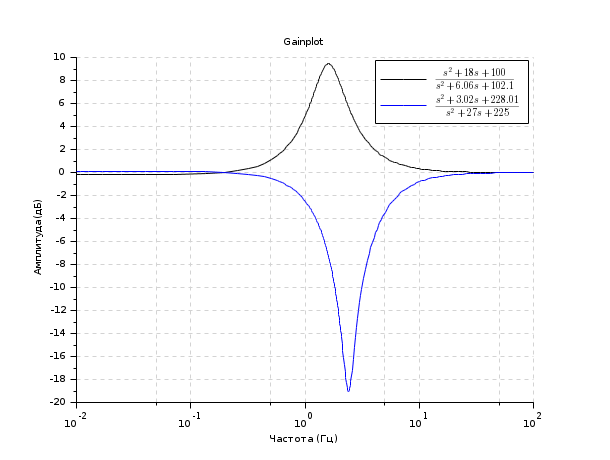Scilab Home page | Wiki | Bug tracker | Forge | Mailing list archives | ATOMS | File exchange
Change language to: English - Français - Português - 日本語 -

See the recommended documentation of this function

Справка Scilab >> CACSD > Plot and display > gainplot

# gainplot

magnitude plot

### Calling Sequence

gainplot(sl,fmin,fmax [,step] [,comments] )
gainplot(frq, repf [,comments])

### Arguments

sl

list (syslin SIMO linear system).

fmin,fmax

real scalars (frequency interval).

step

real (discretization step (logarithmic scale))

string

frq

matrix (row by row frequencies)

db,phi

matrices (magnitudes and phases corresponding to frq)

repf

complex matrix. One row for each frequency response.

### Description

Same as bode but plots only the magnitude.

### Examples

s=poly(0,'s')
h1=syslin('c',(s^2+2*0.9*10*s+100)/(s^2+2*0.3*10.1*s+102.01))
h2=syslin('c',(s^2+2*0.1*15.1*s+228.01)/(s^2+2*0.9*15*s+225))
clf();gainplot([h1;h2],0.01,100,..
["$\frac{s^2+18 s+100}{s^2+6.06 s+102.1}$";
"$\frac{s^2+3.02 s+228.01}{s^2+27 s+225}$"])
title('Gainplot')• bode — Bode plot
• black — Black-Nichols diagram of a linear dynamical system
• nyquist — nyquist plot
• repfreq — frequency response
• g_margin — gain margin and associated crossover frequency
• datatips — Tool for placing and editing tips along the plotted curves.